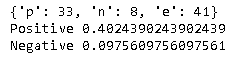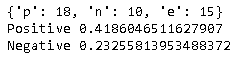# [PYTHON] Let's analyze the questionnaire survey data [4th: Sentiment analysis]

This time, I would like to challenge sentiment analysis using the response data of the questionnaire. By performing sentiment analysis, it is possible to judge whether the respondent's attitude is positive or negative from the words in the free answer.

This time as well, we will use the free answer data on "Why you want to use the electronic version of the medicine notebook" from the URL below.

We conducted an "Awareness Survey on Electronic Medication Notebook" https://www.nicho.co.jp/corporate/newsrelease/11633/

① First, import the library. This time I will use janome.

``````import csv
from janome.tokenizer import Tokenizer
``````

(2) Next, download and load the "Japanese Evaluation Polar Dictionary". This is a word dictionary used to judge words, for example, "honesty" is positive, "bearish" is negative, and about 8,500 expressions are associated with positive or negative (below). URL reference).

http://www.cl.ecei.tohoku.ac.jp/index.php?Open%20Resources%2FJapanese%20Sentiment%20Polarity%20Dictionary

``````! curl http://www.cl.ecei.tohoku.ac.jp/resources/sent_lex/pn.csv.m3.120408.trim > pn.csv

np_dic = {}
fp = open("pn.csv", "rt", encoding="utf-8")
name = row
result = row
np_dic[name] = result
if i % 500 == 0: print(i)
``````

(3) Perform morphological analysis on the target data and compare each word with the above dictionary. In the code below, "p" is a positive, "n" is a negative, and "e" is a neutral phrase that is neither, and each is counted to determine the positive or negative degree.

``````df = open("survey3.txt", "rt", encoding="utf-8")

tok = Tokenizer()

res = {"p":0, "n":0, "e":0}
for t in tok.tokenize(text):
bf = t.base_form
if bf in np_dic:
r = np_dic[bf]
if r in res:
res[r] += 1

print(res)
cnt = res["p"] + res["n"] + res["e"]
print("Positive", res["p"] / cnt)
print("Negative", res["n"] / cnt)
``````

The result is as follows. The answer was just about "why you want to use the electronic version of the medicine notebook", and the result is still highly positive.By the way, this survey also investigates "why you don't want to use the electronic version of the medicine notebook". What if we do a similar analysis with this data?The result looks like this. Despite the "reason why I don't want to use it", the positive level exceeds the negative level. Looking at the original answer data, there were some answers such as "Paper notebook is better", and it seems that the above result was obtained. This area is also related to questionnaire design, and it seems that you need to be careful if you are assuming the implementation of text mining.

### Reference site

Let's evaluate all-you-can-read online novels with negative / positive judgment https://news.mynavi.jp/article/zeropython-58/